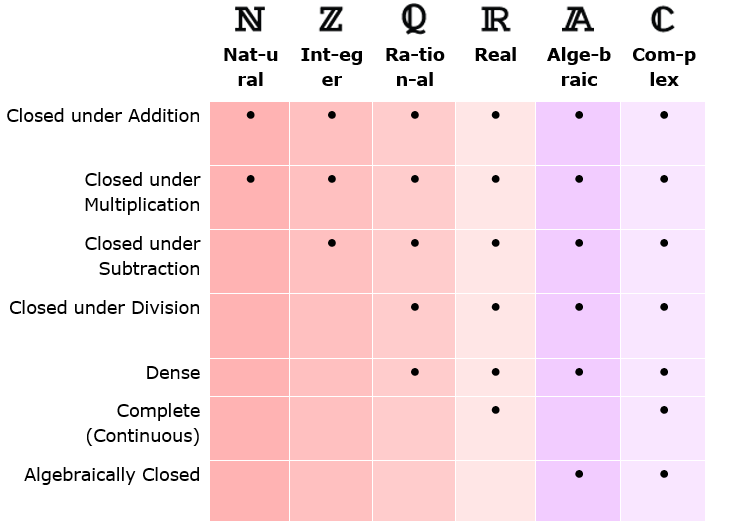Mathematics
Uncountable Sets
15.7k views

 1 Introduction 2 Further Examples 3 Properties of Number Sets 4 FAQs

21 September 2020

## Introduction

A set is uncountable if it contains so many elements that they cannot be put in one-to-one correspondence with the set of natural numbers. In other words, there is no way that one can count off all elements in the set in such a way that, even though the counting will take forever, you will get to any particular element in a finite amount of time.

Uncountable is in contrast to countably infinite or countable.

The uncountability of a set is closely related to its cardinal number: a set is uncountable if its cardinal number is larger than that of the set of all natural numbers.

For example, the set of real numbers between 0 and 1 is an uncountable set because no matter what, you'll always have at least one number that is not included in the set. This set does not have a one-to-one correspondence with the set of natural numbers. The proof of this involves creating an infinite list of numbers between 0 and 1 such as this.

 $$0.12348…$$ $$0.34897…$$ $$0.98789…$$ $$0.43238…$$ $$0.55349...$$

No matter what kind of list you create, there will always be a number that is not in the list. This is found by using Cantor's diagonal argument, where you create a new number by taking the diagonal components of the list and adding 1 to each. So, you take the first place after the decimal in the first number and add one to it. You get $$1 + 1 = 2.$$ Then you take the second place after the decimal in the second number and add 1 to it $$(4 + 1 = 5).$$ And so on to get your new number:

$$0.25840...$$

(When your number is a $$9,$$ you get $$0$$ when adding a $$1$$)

## Further Examples of Uncountable Sets

As we have already seen for countable sets, the concept of countability and cardinality will be explained through examples:

1. Rational Numbers (https://www.cuemath.com/numbers/rational-numbers/)

2. Irrational Numbers (https://www.cuemath.com/numbers/irrational-numbers/)

3. Real Numbers (https://www.cuemath.com/numbers/real-numbers/)

4. Complex Numbers (https://www.cuemath.com/numbers/complex-numbers/)

5. Imaginary Numbers

## Properties of the Number Sets1. Closed under addition (multiplication, subtraction, division) means the sum (product, difference, quotient) of any two numbers in the set is also in the set.

2. Dense: Between any two numbers there is another number in the set.

3. Continuous with no gaps. Every sequence that keeps getting closer together will converge to a limit in the set.

4. Every polynomial with coefficients in the set has a root in the set.

## What is a countable set?

A countable set is a set of numbers that can have a one to one mapping with the set of natural numbers i.e. are either finite or countably infinite.

## What is an uncountable set?

An uncountable set is a set of numbers that don’t have a one to one mapping with the set of natural numbers i.e. they consists of infinite numbers.

## What are a few known examples of a countable set?

Examples of countable set include:

1. Natural Numbers
2. Even Numbers
3. Odd Numbers
4. Whole Numbers
5. Integers
6. Positive Integers
7. Negative Integers, etc.

## What are a few known examples of an uncountable set?

Examples of uncountable set include:

1. Rational Numbers
2. Irrational Numbers
3. Real Numbers
4. Complex Numbers
5. Imaginary Numbers, etc.